Related Articles

# Properties of Determinants – Class 12 Maths

• Last Updated : 17 Dec, 2020

We can see there are 8 important properties of determinants which include Scalar multiple property, Transpose of a determinant (Reflection property) , Row/Column Interchange (Switching Property), Determinant after row operation of adding a proportion of a row to another, Duplicate row determinant (Repetition Property), If all elements of any column or row are zero, then the determinant is zero (All zero property), If all the (sum property) elements of a row or columns in a determinant are expressed as a summation of two or more numbers, then the determinant can be broken down as a sum of corresponding smaller determinants, Determinant of upper triangular or lower triangular matrix (Diagonal Property). All the determinant properties have been covered below in a detailed way along with solved examples.

### Scalar Multiple Property

If any row or column of a determinant, is multiplied by any scalar value, that is, a non-zero constant, the entire determinant gets multiplied by the same scalar, that is, if any row or column is multiplied by constant k, the determinant value gets multiplied by k. Constants may be any real number.

Example 1:Now, on multiplying by k =  1/2, first row,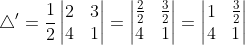det(Δ’) = 1(1) – 4(3/2) = -5

Therefore det(Δ’) = 1/2 det(Δ)
⇒ det(Δ’) = k det(Δ)

Example 2: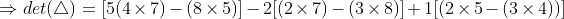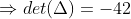Now, on multiplying by k =  , first column,
2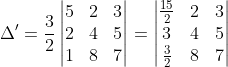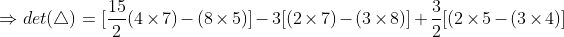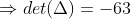Therefore det(Δ’) = 3/2  det(Δ)

⇒ det(Δ’) = k det(Δ)

### Transpose of a determinant (Reflection property)

Transpose refers to the operations of interchanging rows and columns of the determinant. The rows become columns and columns become rows in order. It is denoted by |AT|, for any determinant |A|.
The property says determinant remains unchanged on its transpose, that is, |AT| = |A|.

Example 1: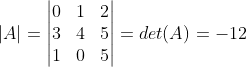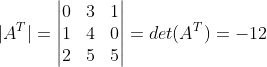⇒ det(A) =  det(AT)

Example 2: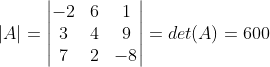### Row/Column Interchange (Switching Property)

If we interchange any two rows/columns of the determinant, the magnitude (that is, the Sign) changes, but the determinant value remains the same.
Now determinant value = (-1)number of exchanges

Example 1: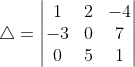If we interchange C1 and C3, denoted by C1↔ C3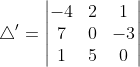det (Δ) = -det(Δ’)
If we again interchange R1 and R2, denoted by R1↔ R2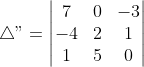det(Δ”) = -det(Δ’)
= det (Δ)

Example 2: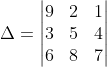Performing a row operation, we get,

If we interchange R1 and R2, denoted by R1 ↔ R2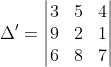det(Δ) = – det(Δ’)
If we again interchange R2 and R3, denoted by R2 ↔ R3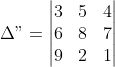det(Δ”) = – det(Δ’)
=  det(Δ)

### Determinant after row operation of adding a proportion of a row to another

If a multiple of one row is added to another row of determinant, its values remain unchanged.

Example 1: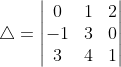det (Δ) = 1 + (-26) = -25

R1 ⇠ R1 + 2R2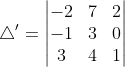det (Δ’) = -6 + (-7)(-1) + (-26)
= -6 + 7 – 26
= -25
det (Δ) = det (Δ’)

Example 2: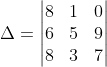det(Δ) = [8{(5 × 7) – (9 × 3)} – 6{(1 × 7) – (0 × 3)} + 8{(1 × 9) – (5 × 0)}]
det(Δ) = 94

R3 ⇢ R3 + 2R2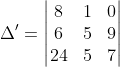det(Δ’) =  [8{(5 × 7) – (9 × 5)} – 6{(1 × 7) – (0 × 5)} + 24{(1 × 9) – (5 × 0)}]
det(Δ’) = 94

det (Δ) = det (Δ’)

### Duplicate row determinant (Repetition Property)

If any pair of rows or columns of a determinant are exactly identical or proportion by same amount, then the determinant is zero

Example 1: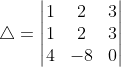det(Δ) = [1{(2 × 0) – (-8 × 3) – 1{(2 × 0) – (-8 × 3)} + 4{(2 × 3) – (2 × 3)}]

det(Δ) = 0 (since R1 and R2 are identical)

Example 2: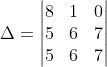det(Δ) = [8{(6 × 7) – (6 × 7)} – 5{(1 × 7) – (6 × 0)} + 5{(1 × 7) – (6 × 0)}]

det(Δ) = 0 (since R2 and R3 are identical)

### All Zero Property

If all elements of any column or row are zero, then the determinant is zero

Example 1: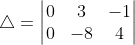det(Δ) = 0 (since C1⇢0)

Example 2: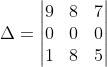det(Δ) = 0 (since R2 ⇢0)

### Sum Property

If all the elements of a row or columns in a determinant are expressed as a summation of two or more numbers, then the determinant can be broken down as a sum of corresponding smaller determinants.### Diagonal Property

If all the elements in the determinant above or below the diagonal are zero, then the determinant is a product of diagonal elements

Example 1: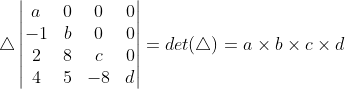Example 2: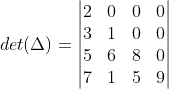det(Δ) = 2×1×8×9 = 144

My Personal Notes arrow_drop_up